`原文链接：http://www.ruanyifeng.com/blog/2017/02/fp-tutorial.html`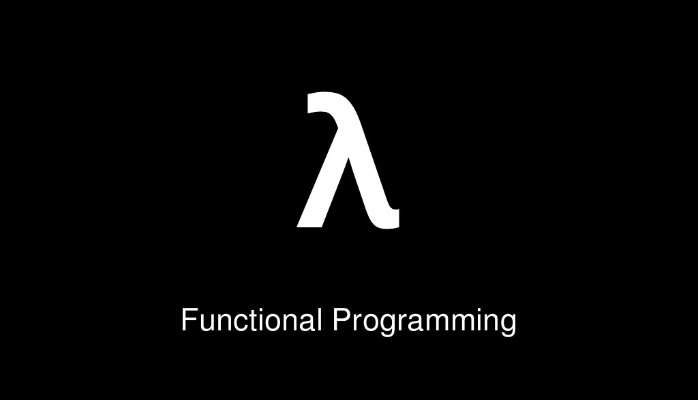• 与面向对象编程（Object-oriented programming）和过程式编程（Procedural programming）并列的编程范式。
• 最主要的特征是，函数是第一等公民
• 强调将计算过程分解成可复用的函数，典型例子就是`map`方法和`reduce`方法组合而成 MapReduce 算法
• 只有纯的、没有副作用的函数，才是合格的函数。## 一、范畴论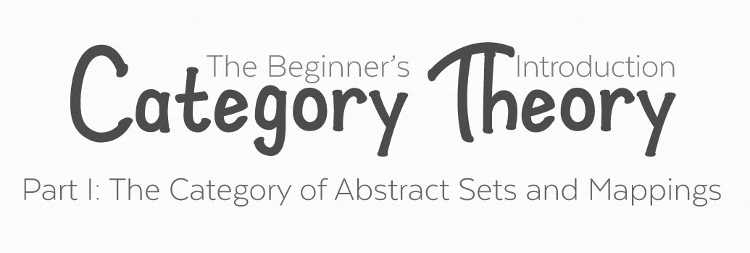### 1.1 范畴的概念

“范畴就是使用箭头连接的物体。”（In mathematics, a category is an algebraic structure that comprises “objects” that are linked by “arrows”. ）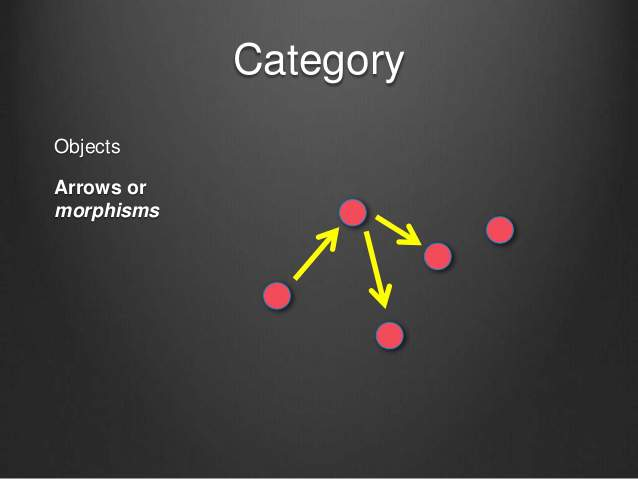• 所有成员是一个集合
• 变形关系是函数

### 1.3 范畴与容器

• 值（value）
• 值的变形关系，也就是函数。

``````class Category {
constructor(val) {
this.val = val;
}

return x + 1;
}
}
``````

## 二、函数的合成与柯里化

### 2.1 函数的合成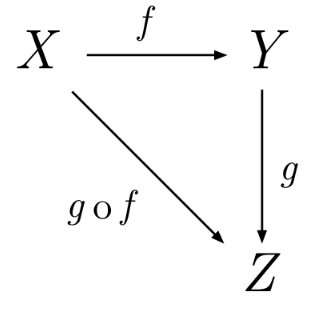``````const compose = function (f, g) {
return function (x) {
return f(g(x));
};
}
````````````compose(f, compose(g, h))
// 等同于
compose(compose(f, g), h)
// 等同于
compose(f, g, h)
``````

### 2.2 柯里化

`f(x)``g(x)`合成为`f(g(x))`，有一个隐藏的前提，就是`f``g`都只能接受一个参数。如果可以接受多个参数，比如`f(x, y)``g(a, b, c)`，函数合成就非常麻烦。

``````// 柯里化之前
return x + y;
}

// 柯里化之后
return function (x) {
return x + y;
};
}

``````

## 三、函子

### 3.1 函子的概念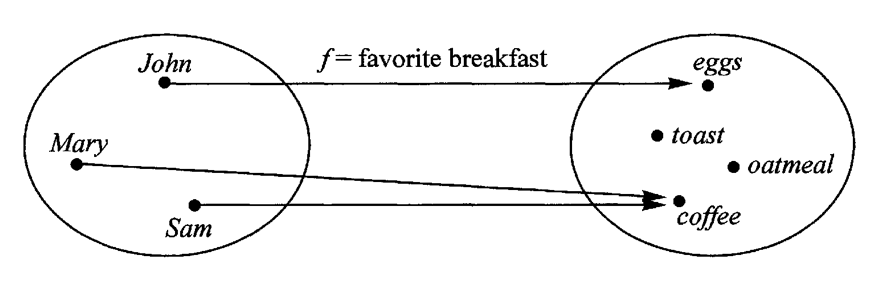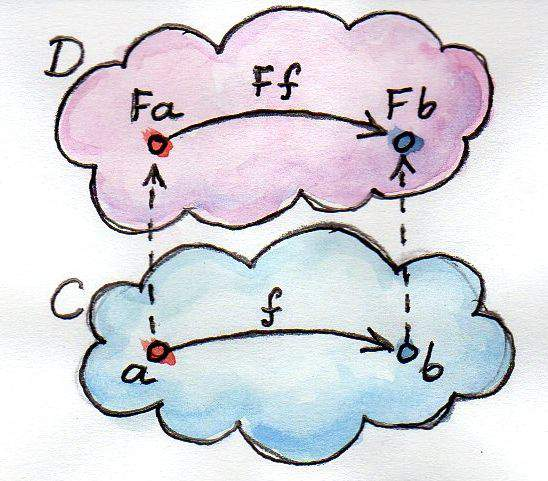### 3.2 函子的代码实现

``````class Functor {
constructor(val) {
this.val = val;
}

map(f) {
return new Functor(f(this.val));
}
}
``````

``````(new Functor(2)).map(function (two) {
return two + 2;
});
// Functor(4)

(new Functor('flamethrowers')).map(function(s) {
return s.toUpperCase();
});
// Functor('FLAMETHROWERS')

(new Functor('bombs')).map(_.concat(' away')).map(_.prop('length'));
// Functor(10)
``````

## 四、of 方法

``````Functor.of = function(val) {
return new Functor(val);
};
``````

``````Functor.of(2).map(function (two) {
return two + 2;
});
// Functor(4)
``````

## 五、Maybe 函子

``````Functor.of(null).map(function (s) {
return s.toUpperCase();
});
// TypeError
``````

Maybe 函子就是为了解决这一类问题而设计的。简单说，它的`map`方法里面设置了空值检查。

``````class Maybe extends Functor {
map(f) {
return this.val ? Maybe.of(f(this.val)) : Maybe.of(null);
}
}
``````

``````Maybe.of(null).map(function (s) {
return s.toUpperCase();
});
// Maybe(null)
``````

## 六、Either 函子

Either 函子内部有两个值：左值（`Left`）和右值（`Right`）。右值是正常情况下使用的值，左值是右值不存在时使用的默认值。

``````class Either extends Functor {
constructor(left, right) {
this.left = left;
this.right = right;
}

map(f) {
return this.right ?
Either.of(this.left, f(this.right)) :
Either.of(f(this.left), this.right);
}
}

Either.of = function (left, right) {
return new Either(left, right);
};
``````

``````var addOne = function (x) {
return x + 1;
};

// Either(5, 7);

// Either(2, null);
``````

Either 函子的常见用途是提供默认值。下面是一个例子。

``````Either
.map(updateField);
``````

Either 函子的另一个用途是代替`try...catch`，使用左值表示错误。

``````function parseJSON(json) {
try {
return Either.of(null, JSON.parse(json));
} catch (e: Error) {
return Either.of(e, null);
}
}
``````

## 七、ap 函子

``````function addTwo(x) {
return x + 2;
}

const A = Functor.of(2);
``````

ap 是 applicative（应用）的缩写。凡是部署了`ap`方法的函子，就是 ap 函子。

``````class Ap extends Functor {
ap(F) {
return Ap.of(this.val(F.val));
}
}
``````

``````Ap.of(addTwo).ap(Functor.of(2))
// Ap(4)
``````

ap 函子的意义在于，对于那些多参数的函数，就可以从多个容器之中取值，实现函子的链式操作。

``````function add(x) {
return function (y) {
return x + y;
};
}

// Ap(5)
``````

``````Ap.of(add(2)).ap(Maybe.of(3));
``````

``````Maybe.of(
Maybe.of(
Maybe.of({name: 'Mulburry', number: 8402})
)
)
``````

Monad 函子的作用是，总是返回一个单层的函子。它有一个`flatMap`方法，与`map`方法作用相同，唯一的区别是如果生成了一个嵌套函子，它会取出后者内部的值，保证返回的永远是一个单层的容器，不会出现嵌套的情况。

``````class Monad extends Functor {
join() {
return this.val;
}
flatMap(f) {
return this.map(f).join();
}
}
``````

## 九、IO 操作

I/O 是不纯的操作，普通的函数式编程没法做，这时就需要把 IO 操作写成`Monad`函子，通过它来完成。

``````var fs = require('fs');

return new IO(function() {
});
};

var print = function(x) {
return new IO(function() {
console.log(x);
return x;
});
}
``````

``````readFile('./user.txt')
.flatMap(print)
``````

``````var tail = function(x) {
return new IO(function() {
return x[x.length - 1];
});
}

.flatMap(tail)
.flatMap(print)

// 等同于